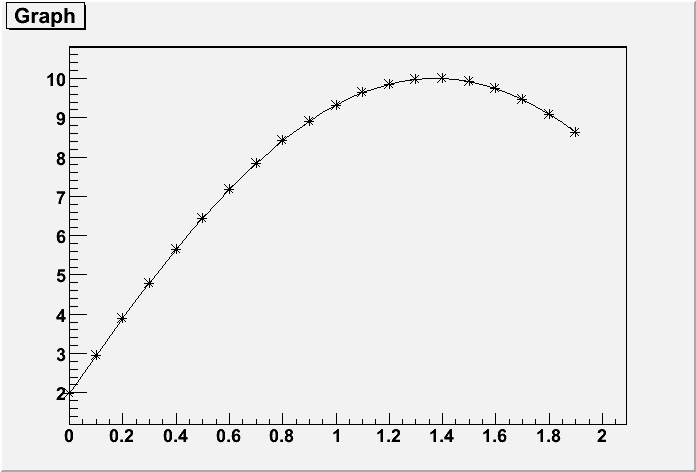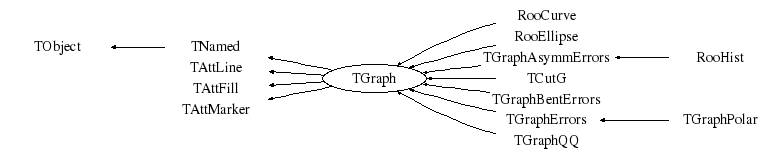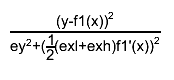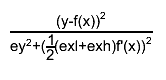# class TGraph: public TNamed, public TAttLine, public TAttFill, public TAttMarker

```
```

## Graph class

A Graph is a graphics object made of two arrays X and Y with npoints each. This class supports essentially two graph categories:
• General case with non equidistant points
• Special case with equidistant points
The various format options to draw a Graph are explained in TGraphPainter::PaintGraph and TGraph::PaintGrapHist These two functions are derived from the HIGZ routines IGRAPH and IGHIST and many modifications.

The picture below gives an example:```{
TCanvas *c1 = new TCanvas("c1","A Simple Graph Example",200,10,700,500);
Double_t x, y;
Int_t n = 20;
for (Int_t i=0;i<n;i++) {
x[i] = i*0.1;
y[i] = 10*sin(x[i]+0.2);
}
gr = new TGraph(n,x,y);
gr->Draw("AC*");
return c1;
}```
```
```

## Function Members (Methods)

public:
protected:
 virtual Double_t** Allocate(Int_t newsize) Double_t** AllocateArrays(Int_t Narrays, Int_t arraySize) virtual void CopyAndRelease(Double_t** newarrays, Int_t ibegin, Int_t iend, Int_t obegin) virtual Bool_t CopyPoints(Double_t** newarrays, Int_t ibegin, Int_t iend, Int_t obegin) Bool_t CtorAllocate() virtual void TObject::DoError(int level, const char* location, const char* fmt, va_list va) const Double_t** ExpandAndCopy(Int_t size, Int_t iend) virtual void FillZero(Int_t begin, Int_t end, Bool_t from_ctor = kTRUE) void TObject::MakeZombie() Double_t** ShrinkAndCopy(Int_t size, Int_t iend) virtual void SwapPoints(Int_t pos1, Int_t pos2) static void SwapValues(Double_t* arr, Int_t pos1, Int_t pos2)

## Data Members

public:
 enum { kClipFrame kNotEditable }; enum TObject::EStatusBits { kCanDelete kMustCleanup kObjInCanvas kIsReferenced kHasUUID kCannotPick kNoContextMenu kInvalidObject }; enum TObject::[unnamed] { kIsOnHeap kNotDeleted kZombie kBitMask kSingleKey kOverwrite kWriteDelete };
protected:
 Color_t TAttFill::fFillColor fill area color Style_t TAttFill::fFillStyle fill area style TList* fFunctions Pointer to list of functions (fits and user) TH1F* fHistogram Pointer to histogram used for drawing axis Color_t TAttLine::fLineColor line color Style_t TAttLine::fLineStyle line style Width_t TAttLine::fLineWidth line width Color_t TAttMarker::fMarkerColor Marker color index Size_t TAttMarker::fMarkerSize Marker size Style_t TAttMarker::fMarkerStyle Marker style Int_t fMaxSize !Current dimension of arrays fX and fY Double_t fMaximum Maximum value for plotting along y Double_t fMinimum Minimum value for plotting along y TString TNamed::fName object identifier Int_t fNpoints Number of points <= fMaxSize TString TNamed::fTitle object title Double_t* fX [fNpoints] array of X points Double_t* fY [fNpoints] array of Y points

## Class Charts## Function documentation

TGraph()
``` Graph default constructor.
```
TGraph(Int_t n)
``` Constructor with only the number of points set
the arrsys x and y will be set later
```
TGraph(Int_t n, const Int_t *x, const Int_t *y)
``` Graph normal constructor with ints.
```
TGraph(Int_t n, const Float_t *x, const Float_t *y)
``` Graph normal constructor with floats.
```
TGraph(Int_t n, const Double_t *x, const Double_t *y)
``` Graph normal constructor with doubles.
```
TGraph(const TGraph &gr)
``` Copy constructor for this graph
```
TGraph& operator=(const TGraph& )
``` Equal operator for this graph
```
TGraph(const TVectorF &vx, const TVectorF &vy)
``` Graph constructor with two vectors of floats in input
A graph is build with the X coordinates taken from vx and Y coord from vy
The number of points in the graph is the minimum of number of points
in vx and vy.
```
TGraph(const TVectorD &vx, const TVectorD &vy)
``` Graph constructor with two vectors of doubles in input
A graph is build with the X coordinates taken from vx and Y coord from vy
The number of points in the graph is the minimum of number of points
in vx and vy.
```
TGraph(const TH1 *h)
``` Graph constructor importing its parameters from the TH1 object passed as argument
```
TGraph(const TF1 *f, Option_t *option)
``` Graph constructor importing its parameters from the TF1 object passed as argument
if option =="" (default), a TGraph is created with points computed
at the fNpx points of f.
if option =="d", a TGraph is created with points computed with the derivatives
at the fNpx points of f.
if option =="i", a TGraph is created with points computed with the integral
at the fNpx points of f.
if option =="I", a TGraph is created with points computed with the integral
at the fNpx+1 points of f and the integral is normalized to 1.
```
TGraph(const char *filename, const char *format, Option_t *)
``` Graph constructor reading input from filename
filename is assumed to contain at least two columns of numbers
the string format is by default "%lg %lg"
```
~TGraph()
``` Graph default destructor.
```
Double_t** AllocateArrays(Int_t Narrays, Int_t arraySize)
``` Allocate arrays.
```
void Apply(TF1* f)
``` Apply function f to all the data points
f may be a 1-D function TF1 or 2-d function TF2
The Y values of the graph are replaced by the new values computed
using the function
```
void Browse(TBrowser* b)
``` Browse
```
Double_t Chisquare(const TF1* f1) const
``` Return the chisquare of this graph with respect to f1.
The chisquare is computed as the sum of the quantity below at each point:$#frac{(y-f1(x))^{2}}{ey^{2}+(#frac{1}{2}(exl+exh)f1'(x))^{2}}$
where x and y are the graph point coordinates and f1'(x) is the derivative of function f1(x).
This method to approximate the uncertainty in y because of the errors in x, is called
"effective variance" method.
In case of a pure TGraph, the denominator is 1.
In case of a TGraphErrors or TGraphAsymmErrors the errors are taken
into account.
```
Bool_t CompareArg(const TGraph* gr, Int_t left, Int_t right)
``` Return kTRUE if point number "left"'s argument (angle with respect to positive
x-axis) is bigger than that of point number "right". Can be used by Sort.
```
Bool_t CompareX(const TGraph* gr, Int_t left, Int_t right)
``` Return kTRUE if fX[left] > fX[right]. Can be used by Sort.
```
Bool_t CompareY(const TGraph* gr, Int_t left, Int_t right)
``` Return kTRUE if fY[left] > fY[right]. Can be used by Sort.
```
Bool_t CompareRadius(const TGraph* gr, Int_t left, Int_t right)
``` Return kTRUE if point number "left"'s distance to origin is bigger than
that of point number "right". Can be used by Sort.
```
void ComputeRange(Double_t& xmin, Double_t& ymin, Double_t& xmax, Double_t& ymax) const
``` Compute the x/y range of the points in this graph
```
void CopyAndRelease(Double_t** newarrays, Int_t ibegin, Int_t iend, Int_t obegin)
``` Copy points from fX and fY to arrays and arrays
or to fX and fY if arrays == 0 and ibegin != iend.
If newarrays is non null, replace fX, fY with pointers from newarrays[0,1].
Delete newarrays, old fX and fY
```
Bool_t CopyPoints(Double_t** newarrays, Int_t ibegin, Int_t iend, Int_t obegin)
``` Copy points from fX and fY to arrays and arrays
or to fX and fY if arrays == 0 and ibegin != iend.
```

``` In constructors set fNpoints than call this method.
Return kFALSE if the graph will contain no points.
```
void Draw(Option_t* chopt = "")
``` Draw this graph with its current attributes.

Options to draw a graph are described in TGraphPainter::PaintGraph
```

``` Compute distance from point px,py to a graph.

Compute the closest distance of approach from point px,py to this line.
The distance is computed in pixels units.
```
void DrawGraph(Int_t n, const Int_t *x, const Int_t *y, Option_t *option)
``` Draw this graph with new attributes.
```
void DrawGraph(Int_t n, const Float_t *x, const Float_t *y, Option_t *option)
``` Draw this graph with new attributes.
```
void DrawGraph(Int_t n, const Double_t *x, const Double_t *y, Option_t *option)
``` Draw this graph with new attributes.
```
void DrawPanel()
``` Display a panel with all graph drawing options.
```
Double_t Eval(Double_t x, TSpline* spline = 0, Option_t* option = "") const
``` Interpolate points in this graph at x using a TSpline
-if spline==0 and option="" a linear interpolation between the two points
close to x is computed. If x is outside the graph range, a linear
extrapolation is computed.
-if spline==0 and option="S" a TSpline3 object is created using this graph
and the interpolated value from the spline is returned.
the internally created spline is deleted on return.
-if spline is specified, it is used to return the interpolated value.
```
void ExecuteEvent(Int_t event, Int_t px, Int_t py)
``` Execute action corresponding to one event.

This member function is called when a graph is clicked with the locator

If Left button clicked on one of the line end points, this point
follows the cursor until button is released.

if Middle button clicked, the line is moved parallel to itself
until the button is released.
```
void Expand(Int_t newsize)
``` If array sizes <= newsize, expand storage to 2*newsize.
```
void Expand(Int_t newsize, Int_t step)
``` If graph capacity is less than newsize points then make array sizes
equal to least multiple of step to contain newsize points.
Returns kTRUE if size was altered
```
Double_t ** ExpandAndCopy(Int_t size, Int_t iend)
``` if size > fMaxSize allocate new arrays of 2*size points
and copy oend first points.
Return pointer to new arrays.
```
void FillZero(Int_t begin, Int_t end, Bool_t from_ctor = kTRUE)
``` Set zero values for point arrays in the range [begin, end)
Should be redefined in descendant classes
```
TObject * FindObject(const char *name)
``` Search object named name in the list of functions
```
TObject * FindObject(const TObject *obj)
``` Search object obj in the list of functions
```
Int_t Fit(const char *fname, Option_t *option, Option_t *, Axis_t xmin, Axis_t xmax)
``` Fit this graph with function with name fname.

interface to TGraph::Fit(TF1 *f1...

fname is the name of an already predefined function created by TF1 or TF2
Predefined functions such as gaus, expo and poln are automatically
created by ROOT.
fname can also be a formula, accepted by the linear fitter (linear parts divided
by "++" sign), for example "x++sin(x)" for fitting "*x+*sin(x)"
```
Int_t Fit(TF1 *f1, Option_t *option, Option_t *, Axis_t rxmin, Axis_t rxmax)
``` Fit this graph with function f1.

f1 is an already predefined function created by TF1.
Predefined functions such as gaus, expo and poln are automatically
created by ROOT.

The list of fit options is given in parameter option.
option = "W" Set all weights to 1; ignore error bars
= "U" Use a User specified fitting algorithm (via SetFCN)
= "Q" Quiet mode (minimum printing)
= "V" Verbose mode (default is between Q and V)
= "B" Use this option when you want to fix one or more parameters
and the fitting function is like "gaus","expo","poln","landau".
= "R" Use the Range specified in the function range
= "N" Do not store the graphics function, do not draw
= "0" Do not plot the result of the fit. By default the fitted function
is drawn unless the option"N" above is specified.
= "+" Add this new fitted function to the list of fitted functions
(by default, any previous function is deleted)
= "C" In case of linear fitting, not calculate the chisquare
(saves time)
= "F" If fitting a polN, switch to minuit fitter
= "ROB" In case of linear fitting, compute the LTS regression
coefficients (robust(resistant) regression), using
the default fraction of good points
"ROB=0.x" - compute the LTS regression coefficients, using
0.x as a fraction of good points

When the fit is drawn (by default), the parameter goption may be used
to specify a list of graphics options. See TGraph::Paint for a complete
list of these options.

In order to use the Range option, one must first create a function
with the expression to be fitted. For example, if your graph
has a defined range between -4 and 4 and you want to fit a gaussian
only in the interval 1 to 3, you can do:
TF1 *f1 = new TF1("f1","gaus",1,3);
graph->Fit("f1","R");

Who is calling this function:

Note that this function is called when calling TGraphErrors::Fit
or TGraphAsymmErrors::Fit ot TGraphBentErrors::Fit
see the discussion below on the errors calulation.

Linear fitting:

When the fitting function is linear (contains the "++" sign) or the fitting
function is a polynomial, a linear fitter is initialised.
To create a linear function, use the following syntaxis: linear parts
separated by "++" sign.
Example: to fit the parameters of "*x + *sin(x)", create a
TF1 *f1=new TF1("f1", "x++sin(x)", xmin, xmax);
For such a TF1 you don't have to set the initial conditions
Going via the linear fitter for functions, linear in parameters, gives a considerable

Setting initial conditions:

Parameters must be initialized before invoking the Fit function.
The setting of the parameter initial values is automatic for the
predefined functions : poln, expo, gaus, landau. One can however disable
this automatic computation by specifying the option "B".
You can specify boundary limits for some or all parameters via
f1->SetParLimits(p_number, parmin, parmax);
if parmin>=parmax, the parameter is fixed
Note that you are not forced to fix the limits for all parameters.
For example, if you fit a function with 6 parameters, you can do:
func->SetParameters(0,3.1,1.e-6,0.1,-8,100);
func->SetParLimits(4,-10,-4);
func->SetParLimits(5, 1,1);
With this setup, parameters 0->3 can vary freely
Parameter 4 has boundaries [-10,-4] with initial value -8
Parameter 5 is fixed to 100.

Fit range:

The fit range can be specified in two ways:
- specify rxmax > rxmin (default is rxmin=rxmax=0)
- specify the option "R". In this case, the function will be taken
instead of the full graph range.

Changing the fitting function:

By default the fitting function GraphFitChisquare is used.
To specify a User defined fitting function, specify option "U" and
call the following functions:
TVirtualFitter::Fitter(mygraph)->SetFCN(MyFittingFunction)
where MyFittingFunction is of type:
extern void MyFittingFunction(Int_t &npar, Double_t *gin, Double_t &f, Double_t *u, Int_t flag);

How errors are used in the chisquare function (see TFitter GraphFitChisquare)//   Access to the fit results

In case of a TGraphErrors object, ex, the error along x,  is projected
along the y-direction by calculating the function at the points x-exlow and
x+exhigh.

The chisquare is computed as the sum of the quantity below at each point:$#frac{(y-f(x))^{2}}{ey^{2}+(#frac{1}{2}(exl+exh)f'(x))^{2}}$

where x and y are the point coordinates, and f'(x) is the derivative of function f(x).

In case the function lies below (above) the data point, ey is ey_low (ey_high).

thanks to Andy Haas (haas@yahoo.com) for adding the case with TGraphasymmerrors
University of Washington

The approach used to approximate the uncertainty in y because of the
errors in x, is to make it equal the error in x times the slope of the line.
The improvement, compared to the first method (f(x+ exhigh) - f(x-exlow))/2
is of (error of x)**2 order. This approach is called "effective variance method".
This improvement has been made in version 4.00/08 by Anna Kreshuk.

NOTE:
1) By using the "effective variance" method a simple linear regression
becomes a non-linear case, which takes several iterations
instead of 0 as in the linear case .

2) The effective variance technique assumes that there is no correlation
between the x and y coordinate .

Note, that the linear fitter doesn't take into account the errors in x. If errors
in x are important, go through minuit (use option "F" for polynomial fitting).

3) When fitting a TGraph (ie no errors associated to each point),
a correction is applied to the errors on the parameters with the following
formula:
errorp *= sqrt(chisquare/(ndf-1))

Associated functions:

One or more object (typically a TF1*) can be added to the list
of functions (fFunctions) associated to each graph.
When TGraph::Fit is invoked, the fitted function is added to this list.
Given a graph gr, one can retrieve an associated function
with:  TF1 *myfunc = gr->GetFunction("myfunc");

If the graph is made persistent, the list of
associated functions is also persistent. Given a pointer (see above)
to an associated function myfunc, one can retrieve the function/fit
parameters with calls such as:
Double_t chi2 = myfunc->GetChisquare();
Double_t par0 = myfunc->GetParameter(0); //value of 1st parameter
Double_t err0 = myfunc->GetParError(0);  //error on first parameter

Fit Statistics

You can change the statistics box to display the fit parameters with
the TStyle::SetOptFit(mode) method. This mode has four digits.
mode = pcev  (default = 0111)
v = 1;  print name/values of parameters
e = 1;  print errors (if e=1, v must be 1)
c = 1;  print Chisquare/Number of degress of freedom
p = 1;  print Probability

For example: gStyle->SetOptFit(1011);
prints the fit probability, parameter names/values, and errors.
You can change the position of the statistics box with these lines
(where g is a pointer to the TGraph):

Root > TPaveStats *st = (TPaveStats*)g->GetListOfFunctions()->FindObject("stats")
Root > st->SetX1NDC(newx1); //new x start position
Root > st->SetX2NDC(newx2); //new x end position

The function return the status of the fit (fitResult) in the following form
fitResult = migradResult + 10*minosResult + 100*hesseResult + 1000*improveResult
The fitResult is 0 is the fit is OK.
The fitResult is negative in case of an error not connected with the fit.
```
void FitPanel()
``` Display a GUI panel with all graph fit options.

See class TFitEditor for example
```

``` Return graph correlation factor
```

``` Return covariance of vectors x,y
```
Double_t GetMean(Int_t axis = 1) const
``` Return mean value of X (axis=1)  or Y (axis=2)
```
Double_t GetRMS(Int_t axis = 1) const
``` Return RMS of X (axis=1)  or Y (axis=2)
```
Double_t GetErrorX(Int_t bin) const
``` This function is called by GraphFitChisquare.
It always returns a negative value. Real implementation in TGraphErrors
```
Double_t GetErrorY(Int_t bin) const
``` This function is called by GraphFitChisquare.
It always returns a negative value. Real implementation in TGraphErrors
```
Double_t GetErrorXhigh(Int_t bin) const
``` This function is called by GraphFitChisquare.
It always returns a negative value. Real implementation in TGraphErrors
and TGraphAsymmErrors
```
Double_t GetErrorXlow(Int_t bin) const
``` This function is called by GraphFitChisquare.
It always returns a negative value. Real implementation in TGraphErrors
and TGraphAsymmErrors
```
Double_t GetErrorYhigh(Int_t bin) const
``` This function is called by GraphFitChisquare.
It always returns a negative value. Real implementation in TGraphErrors
and TGraphAsymmErrors
```
Double_t GetErrorYlow(Int_t bin) const
``` This function is called by GraphFitChisquare.
It always returns a negative value. Real implementation in TGraphErrors
and TGraphAsymmErrors
```
TF1 * GetFunction(const char* name) const
``` Return pointer to function with name.

Functions such as TGraph::Fit store the fitted function in the list of
functions of this graph.
```

``` Returns a pointer to the histogram used to draw the axis
Takes into account the two following cases.
1- option 'A' was specified in TGraph::Draw. Return fHistogram
```
void GetPoint(Int_t i, Double_t& x, Double_t& y) const
``` Get x and y values for point number i.
```

``` Get x axis of the graph.
```

``` Get y axis of the graph.
```
void InitGaus(Double_t xmin = 0, Double_t xmax = 0)
``` Compute Initial values of parameters for a gaussian.
```
void InitExpo(Double_t xmin = 0, Double_t xmax = 0)
``` Compute Initial values of parameters for an exponential.
```
void InitPolynom(Double_t xmin = 0, Double_t xmax = 0)
``` Compute Initial values of parameters for a polynom.
```

``` Insert a new point at the mouse position
```
void LeastSquareFit(Int_t m, Double_t* a, Double_t xmin = 0, Double_t xmax = 0)
``` Least squares lpolynomial fitting without weights.

m     number of parameters
a     array of parameters
first 1st point number to fit (default =0)
last  last point number to fit (default=fNpoints-1)

based on CERNLIB routine LSQ: Translated to C++ by Rene Brun
```
void LeastSquareLinearFit(Int_t n, Double_t& a0, Double_t& a1, Int_t& ifail, Double_t xmin = 0, Double_t xmax = 0)
``` Least square linear fit without weights.

Fit a straight line (a0 + a1*x) to the data in this graph.
ndata:  if ndata<0, fits the logarithm of the graph (used in InitExpo() to set
the initial parameter values for a fit with exponential function.
a0:     constant
a1:     slope
ifail:  return parameter indicating the status of the fit (ifail=0, fit is OK)
xmin, xmax: fitting range

extracted from CERNLIB LLSQ: Translated to C++ by Rene Brun
```
void Paint(Option_t* chopt = "")
``` Draw this graph with its current attributes.
```
void PaintGraph(Int_t npoints, const Double_t* x, const Double_t* y, Option_t* chopt)
``` Draw the (x,y) as a graph.
```
void PaintGrapHist(Int_t npoints, const Double_t* x, const Double_t* y, Option_t* chopt)
``` Draw the (x,y) as a histogram.
```
void PaintStats(TF1* fit)
``` Draw the stats
```
void Print(Option_t* chopt = "") const
``` Print graph values.
```
void RecursiveRemove(TObject* obj)
``` Recursively remove object from the list of functions
```
Int_t RemovePoint(Int_t ipoint)
``` Delete point close to the mouse position
```
Int_t RemovePoint(Int_t ipoint)
``` Delete point number ipoint
```
void SavePrimitive(ostream& out, Option_t* option = "")
``` Save primitive as a C++ statement(s) on output stream out
```
void Set(Int_t n)
``` Set number of points in the graph
Existing coordinates are preserved
New coordinates above fNpoints are preset to 0.
```

``` Return kTRUE if kNotEditable bit is not set, kFALSE otherwise.
```
void SetEditable(Bool_t editable = kTRUE)
``` if editable=kFALSE, the graph cannot be modified with the mouse
by default a TGraph is editable
```
void SetMaximum(Double_t maximum = -1111)
``` Set the maximum of the graph.
```
void SetMinimum(Double_t minimum = -1111)
``` Set the minimum of the graph.
```
void SetPoint(Int_t i, Double_t x, Double_t y)
``` Set x and y values for point number i.
```
void SetTitle(const char* title = "")
``` Set graph title.
```
Double_t ** ShrinkAndCopy(Int_t size, Int_t iend)
``` if size*2 <= fMaxSize allocate new arrays of size points,
copy points [0,oend).
Return newarray (passed or new instance if it was zero
and allocations are needed)
```
void Sort(Bool_t (*greaterfunc)(const TGraph*, Int_t, Int_t) /*=TGraph::CompareX()*/, Bool_t ascending /*=kTRUE*/, Int_t low /* =0 */, Int_t high /* =-1111 */)
``` Sorts the points of this TGraph using in-place quicksort (see e.g. older glibc).
To compare two points the function parameter greaterfunc is used (see TGraph::CompareX for an
example of such a method, which is also the default comparison function for Sort). After
the sort, greaterfunc(this, i, j) will return kTRUE for all i>j if ascending == kTRUE, and
kFALSE otherwise.

The last two parameters are used for the recursive quick sort, stating the range to be sorted

Examples:
// sort points along x axis
graph->Sort();
// sort points along their distance to origin

Bool_t CompareErrors(const TGraph* gr, Int_t i, Int_t j) {
const TGraphErrors* ge=(const TGraphErrors*)gr;
return (ge->GetEY()[i]>ge->GetEY()[j]); }
// sort using the above comparison function, largest errors first
graph->Sort(&CompareErrors, kFALSE);
```
void Streamer(TBuffer& b)
``` Stream an object of class TGraph.
```
void SwapPoints(Int_t pos1, Int_t pos2)
``` Swap points.
```
void SwapValues(Double_t* arr, Int_t pos1, Int_t pos2)
``` Swap values.
```
void UseCurrentStyle()
``` Set current style settings in this graph
This function is called when either TCanvas::UseCurrentStyle
or TROOT::ForceStyle have been invoked.
```
Int_t Merge(TCollection* list)
``` Adds all graphs from the collection to this graph.
Returns the total number of poins in the result or -1 in case of an error.
```
void Zero(Int_t& k, Double_t AZ, Double_t BZ, Double_t E2, Double_t& X, Double_t& Y, Int_t maxiterations)
``` Find zero of a continuous function.
This function finds a real zero of the continuous real
function Y(X) in a given interval (A,B). See accompanying
notes for details of the argument list and calling sequence
```
Double_t ** Allocate(Int_t newsize)

`{ return fFunctions; }`

`{return fMaxSize;}`

`{return fNpoints;}`
Double_t * GetX()
`{return fX;}`
Double_t * GetY()
`{return fY;}`

`{return 0;}`

`{return 0;}`

`{return 0;}`

`{return 0;}`

`{return 0;}`

`{return 0;}`

`{return 0;}`

`{return 0;}`

`{return 0;}`

`{return 0;}`

`{return fMaximum;}`

`{return fMinimum;}`

`{return !TestBit(kNotEditable);}`
void SetHistogram(TH1* h)
`{fHistogram = (TH1F*)h;}`

Author: Rene Brun, Olivier Couet 12/12/94
Last change: root/graf:\$Id: TGraph.h 24105 2008-06-02 18:53:37Z brun \$
Last generated: 2008-06-25 08:46